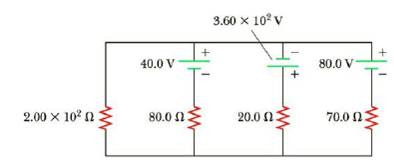Chapter 18, Problem 23P

Chapter
Section
Textbook Problem

In the circuit of Figure P18.23, determine (a) the current in each resistor, (b) the potential difference across the 2.00 × 102-Ω resistor, and (c) the power delivered by each battery.Figure P18.23

(a)

To determine
The current in each resistor I1,I2,I3,I4

Explanation

Given Info:

Explanation:

Resistance R1 is 2.00×102Ω , Resistance R2 is 80.0Ω , Resistance R3 is 20.0Ω , Resistance R4 is 70.0Ω , Voltage ε1 is 40.0V , Voltage ε2 is 3.60×102V , and Voltage ε3 is 80.0V .

The below figure shows the path of current in the circuit

• ε1,ε2,ε3 is voltage in the circuits
• R1,R2,R3,R4 is corresponding resistance in the circuits
• I1,I2,I3,I4 is corresponding current in the circuits

Apply Kirchhoff’s voltage law

From the left loop dbacd,

ε1R1I1+R2I2=0

Substitute, 2.00×102Ω for R1 , 80.0Ω for R2 , 40.0V for ε1 , in the above equation to find the expression for I2

80.0ΩI2=40.0V+2.00×102ΩI1I2=40.0V80.0Ω+2.00×102Ω80.0ΩI1=0.5A+2.5I1 (I)

From the middle loop cefdc,

ε1+ε2R2I2R3I3=0

Substitute, 80.0Ω for R2 , 20.0Ω for R3 40.0V for ε1 , 3.60×102V for ε2 in the above equation to find the expression for I3

0=40.0V+3.60×102V80.0ΩI220.0ΩI320.0ΩI3=40.0V+3.60×102V80.0ΩI2I3=40.0V20.0Ω+3.60×102V20.0Ω80.0Ω20.0ΩI2=2.00A+18.0A4I2=20.0A4I2 (II)

From the right loop ghbag

ε3+R4I4R1I1=0

Substitute, 2

(b)

To determine
The potential difference (V1) across in 2.00×102Ω is R1

(c)

To determine
The Power delivered by each battery.

Still sussing out bartleby?

Check out a sample textbook solution.

See a sample solution

The Solution to Your Study Problems

Bartleby provides explanations to thousands of textbook problems written by our experts, many with advanced degrees!

Get Started